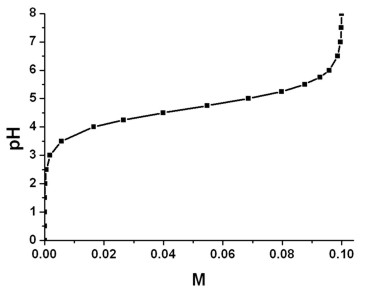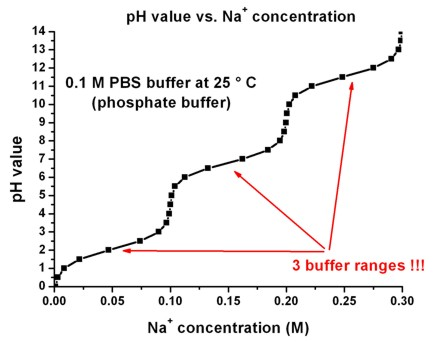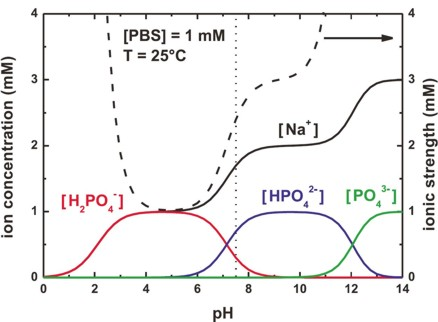nextnano.com  nextnano³  Download | Search | Copyright | Publications  * password protected nextnano³ softwarebuffer-solutions

# Buffer solutions: To control pH values in electrolytes.

```!------------------------------------------------------! \$buffer-solutions                          optional    !  buffer-name             character         required    !  number-of-ions          integer           required    !  ion-valency             double_array      required    !  ion-name-1              character         required    !  ion-name-2              character         required    !  ion-name-3              character         optional    ! ```(only necessary for some buffers, e.g. PBS)```  ion-name-4              character         optional    ! ```(only necessary for some buffers, e.g. PBS)```  pKa                     double_array      required    ! ```pKa at 25° C ( = 298.15 K)```  dpKa_dT                 double_array      required    ! ```d pKa / d T```  z_acid                  double_array      required    ! ```charge on the conjugate acid species``` \$end_buffer-solutions                      optional    ! !------------------------------------------------------!```

## Syntax

```                                                 ! ```name of buffer```  buffer-name    = ACETATE                        ! ```Acetate (sodium acetate + acetic acid) (CH3COO`-`Na+ + CH3COOH)```                 = HEPES                          ! ```HEPES (C8H18N2O4S)```                 = TRIS                           ! ```TRIS     (C4H11NO3)```                 = MOPS                           ! ```MOPS  (C7H15NO4S)```                 = PBS                            ! ```PBS (phosphate buffer)```  ```

```                                                 ! ```number of ions that are contained in the buffer```  number-of-ions = 2                              ! 2``` for` ACETATE`,``` HEPES```,``` TRIS ```and``` MOPS                 = 4                              ! 4``` for` PBS`

```                                                 ! ```valency of the ions that are contained in the buffer```  ion-valency    = -1d0  +1d0                     ! ```for``` ACETATE```:  (CH3COO)`-`          Na+
```                = +1d0  -1d0                     ! ```for``` TRIS```:        TrisH+                    (OH)`-`
```                = -1d0  +1d0                     ! ```for``` MOPS```:        (C7H14NO4S)`-`` `  Na+
```                = -1d0  -2d0  -3d0  1d0          ! ```for` PBS`:          (H2PO4)```-   ```(HPO4)2`-    `(PO4)3```-    ```Na+
```                                                 ! ```(the number of expected entries corresponds to` number-of-ions`)

```                                                 ! ```name of the ions that are contained in the buffer
```                                                 !                                                  ! ```CH3COOH`  <==> `(CH3COO)`-```` + ```H+```  ion-name-1     = CH3COO^-                       ! ```name of ion no. 1 (for` ACETATE`)```  ion-name-2     = Na^+                           ! ```name of ion no. 2 (for` ACETATE`)
```                                                 !                                                  ! ```TrisH+`     <==> `Tris```   + ```H+           (  "NH3+"```      <==> ```"NH2"`  + `H+       )
```                                                 ! ```Tris` + `H20` <==> `TrisH+``` + ```(OH)`-`       (  "NH2"` + `H20` <==> `"NH3+"``` + ```(OH)`-`  )```  ion-name-1     = TrisH^+                        ! ```name of ion no. 1 (for` TRIS`)```  ion-name-2     = OH^-                           ! ```name of ion no. 2 (for` TRIS`)
```                                                 !                       ```      ("SO3H"` <==> ` "(SO3)`-`" ```     + ```H+)```  ion-name-1     = Mops^-                         ! ```name of ion no. 1 (for` MOPS`)   C7H15NO4S` <==> `(C7H14NO4S)`-```` + ```H+```  ion-name-2     = Na^+                           ! ```name of ion no. 2 (for` MOPS`)
```  ion-name-1     = H2PO4^-                        ! ```name of ion no. 1 (for` PBS`)     NaH2PO4` <==> `(H2PO4)`-```` +   ```Na+```  ion-name-2     = HPO4^2-                        ! ```name of ion no. 2 (for` PBS`)     Na2HPO4` <==> `(HPO4)2`-```` + 2 ```Na+```  ion-name-3     = PO4^3-                         ! ```name of ion no. 3 (for` PBS`)      (HPO4)2`-`` <==> `(PO4)3`-````  +   ```H+```  ion-name-4     = Na^+                           ! ```name of ion no. 4 (for` PBS`) ```  ```

```                                                 ! ```pKa value(s) of buffer reactions```                                                  ! ```One can calculate the pH value from the pKa value and
```                                                 ! ```the concentrations of acid and base (Henderson-Hasselbalch equation):```                                                  !         pH = ```pKa``` + log10 ([base]/[acid])                                                  ! ```Example:` pH = `pKa``` + log10 ([ ```Cl`- ]/[ `HCl ```])                                                  !         ```HCl` + `H20``` <==> ```Cl`- + `H30```+  pKa            = 4.76d0                         ! ```for` ACETATE`: pKa                                      at 25° C ( = 298.15 K)```                 = 7.66d0                         ! ```for` HEPES`:     pKa                                      at 25° C ( = 298.15 K)```                 = 8.06d0                         ! ```for` TRIS`:       pKa                                      at 25° C ( = 298.15 K)```                 = 7.31d0                         ! ```for` MOPS`:       pKa                                      at 25° C ( = 298.15 K)```                 = 2.15d0     7.21d0    12.33d0   ! ```for` PBS`:         pKa,1    pKa,2    pKa,3    at 25° C ( = 298.15 K)```  ```

```                                                 ! ```d pKa / d T value(s) of pKa value(s), i.e. temperature dependence of pKa value(s)```                                                  ! ```(temperature coefficient, i.e. the rate of change of pKa with temperature)```                                                  ! ```If the buffer solution warms up, the pKa value drops.```                                                  ! ```For further details, see``` \$electrolyte```.```  dpKa_dT        = -0.0002d0                      ! ```for` ACETATE`:  d pKa   / d T```                 = -0.014d0                       ! ```for` HEPES`:      d pKa   / d T```                 = -0.028d0                       ! ```for` TRIS`:        d pKa   / d T```                 = -0.011d0                       ! ```for` MOPS`:        d pKa   / d T```                 =  0.0044d0  -0.0028d0  -0.026d0 ! ```for` PBS`:          d pKa,1 / d T      d pKa,2 / d T      d pKa,3 / d T

```                                                 ! ```charge on the conjugate acid species```                                                  ! ```Note:   'conjugate acid' ` + `H20``` <==> ```'conjugate base' ` + `H30```+                                                  ! ```This value enters the equation for the ionic strength dependence
```                                                 ! ```of the pKa value (modified pKa value: pKa' ).```  z_acid         =  0d0                           ! ```for``` ACETATE```:  ` 0` = CH3COOH```                 =  0d0                           ! ```for``` MOPS```:  `    0` = C7H15NO4S```                 = +1d0                           ! ```for``` TRIS```:  `   +1` = TrisH+```                 =  0d0       -1d0       -2d0     ! ```for` PBS`:    `    0` = H3PO4, `-1` = (H2PO4)`-` , `-2` = (HPO4)2`-````  ```

Examples: Acetate, MOPS and PBS (phosphate buffer)

The phosphate buffer is special (and thus more complicated) because it consists of three pKa values (and it thus has four different ions).

```!------------------------------------------------! \$buffer-solutions                                !                                                  !  buffer-name    = ACETATE                        ! ```Acetate (sodium acetate + acetic acid) (CH3COO`-`Na+ + CH3COOH)```  number-of-ions = 2                              ! ```CH3COOH`  <==> `(CH3COO)`-```` + ```H+```  ion-valency    = -1d0 +1d0                      !  ion-name-1     = CH3COO^-                       ! ```(CH3COO)`-````  ion-name-2     = Na^+                           ! ```Na+```  pKa            = 4.76d0                         ! ```pKa at 25° C ( = 298.15 K)```  dpKa_dT        = -0.0002d0                      ! ```d pKa   / d T```  z_acid         = 0d0                            ! ```charge on the conjugate acid species (`0` = CH3COOH)```                                                  !                                                  !  buffer-name    = MOPS                           ! ```MOPS  (C7H15NO4S)  +  NaOH```  number-of-ions = 2                              !  ion-valency    = -1d0  +1d0                     ! ```(C7H14NO4S)`-````  ```Na+``` `````` ion-name-1     = Mops^-                         ! ``` C7H15NO4S` <==> `(C7H14NO4S)`-```` + ```H+```  ion-name-2     = Na^+                           !  pKa            = 7.31d0                         ! ```pKa at 25° C ( = 298.15 K)
```                                                 ! ```(Note: This pKa is thermodynamic value. The working pKa' is 7.20.)```  dpKa_dT        = -0.011d0                       ! ```d pKa   / d T```  z_acid         = 0d0                            ! ```charge on the conjugate acid species (`0` = C7H15NO4S)```                                                  !                                                  !  buffer-name    = PBS                            ! ```PBS (phosphate buffer)```  number-of-ions = 4                              !  ion-valency    = -1d0  -2d0  -3d0  1d0          ! ```(H2PO4)`-`     (HPO4)2`-`     (PO4)3`-`     Na+``` `````` ion-name-1     = H2PO4^-                        ! ```NaH2PO4` <==> `(H2PO4)`-```` +   ```Na+```  ion-name-2     = HPO4^2-                        ! ```Na2HPO4` <==> `(HPO4)2`-```` + 2 ```Na+```  ion-name-3     = PO4^3-                         !  ion-name-4     = Na^+                           !  pKa            = 2.15d0     7.21d0    12.33d0   ! ```pKa,1    pKa,2    pKa,3    at 25° C ( = 298.15 K)```  dpKa_dT        = 0.0044d0  -0.0028d0  -0.026d0  ! ```d pKa   / d T```  z_acid         = 0d0       -1d0       -2d0      ! ```charge on the conjugate acid species (`0` = H3PO4, `-1` = (H2PO4)`-` , `-2` = (HPO4)2`-`)```                                                  ! \$end_buffer-solutions                            ! !------------------------------------------------!  ```

For more details on buffers, please have a look at the excellent book of
R.J. Beynon, J.S. Easterby, "Buffer solutions: The basics", Oxford University Press (1996).

Rob Beynon also provides a web interface "A recipe calculator for thermodynamically correct buffers for pH control" at:``` http://www.liv.ac.uk/buffers/```

Example: Acetate buffer (sodium acetate + acetic acid)

• The following figure shows the pH value as a function of the concentration of the (CH3COO)`-` ions. Note that this number is equal to the concentration of Na+ ions (see equations above).

The concentration of the acetate buffer is 0.1 M, i.e. it consists of
`- `0.1 M CH3COOH (acetic acid) and
`- `0.1 M CH3COO`-`Na+ (sodium acetate).
The temperature was set to 298.15 K ( = 25° C).
The pKa value at 25° C is 4.76. Note that the pKa value depends on temperature and ionic strength. Thus for each pH value a different value of pKa had been calculated self-consistently` => `pKa'.

The nextnano³ calculations were performed by looping over the pH values. Note that the code adds the appropriate concentrations of Cl`-` ions (originating from the acid HCl) and Na+ ions (originating from the base NaOH) automatically.

CH3COO`-`   +   H3O+   +   Cl`-` `  <==>  `CH3COOH   +   H2O   +   Cl`-`
CH3COOH  +   Na+     +   OH`-``  <==>  `CH3COO`-`    +   H2O   +   Na+

The acetate buffer has the best buffering range (i.e. pH = +`-`1) at around pKa = 4.76, i.e. it buffers nicely between pH = 3.76 and pH = 5.76.

Below the pH value of 3, the concentration of (CH3COO)`-` is negligible and only CH3COOH  exists.
Above the pH value of 8, the concentration of CH3COOH  is negligible and only (CH3COO)`-` exists.

pH value vs. [CH3COO`-`]The relation between the pH value, the pKa' value and the concentration of CH3COO`-` ions is governed by the Henderson-Hasselbalch equation.

pH = pKa'  +  log (  [CH3COO`-`] / [CH3COOH] )

The concentration of all ions (not only the buffer ions), the ionic strength, as well as the pKa' value(s) as a function of pH are contained in this file:
`BufferIonConc_vs_pH1D.dat`

If you want to obtain the nextnano³ input file (`Buffer_Acetate.in`) that calculates the above figure, please submit a support ticket.

Details on the calculations:

• `ACETATE `(sodium acetate + acetic acid)

[CH3COOH]  = [ACETATE] / ( 1 + 10pH`-`pKa )
[(CH3COO)`-`] = [CH3COOH] * 10pH`-`pKa`       !  ` Henderson-Hasselbalch equation
[Na+]            = [(CH3COO)`-`]

• `MOPS`

[C7H15NO4S]     = [MOPS] / ( 1 + 10pH`-`pKa )
[(C7H14NO4S)`-`] = [C7H15NO4S] * 10pH`-`pKa`    !  ` Henderson-Hasselbalch equation
[Na+]                 = [(C7H14NO4S)`-`]

(analogous for` HEPES `and``` TRIS```)

• `PBS `(phosphate buffer)

[H3PO4]    = [PBS] / ( 1 + 10pH`-`pKa,1 * ( 1 + 10pH`-`pKa,2  * ( 1 + 10pH`-`pKa,3 ) ) )
[(H2PO4)`-`] = [H3PO4]    * 10pH`-`pKa,1```        !  ```Henderson-Hasselbalch equation
[(HPO4)2`-`] = [(H2PO4)`-`] * 10pH`-`pKa,2```        !  ```Henderson-Hasselbalch equation
[(PO4)3`-`]   = [(HPO4)2`-`] * 10pH`-`pKa,3```        !  ```Henderson-Hasselbalch equation
[Na+]         = (1 * [(H2PO4)`-`] + 2 * [(HPO4)2`-`] + 3 * [(PO4)3`-`]

The following figure shows the pH value as a functions of the Na+ concentration for a PBS buffer. Note that there are three buffer ranges.In this figure, we plot the concentration of the PBS buffer ions, and the resulting ionic strength as a function of pH.If you want to obtain the nextnano³ input file (`Buffer_PBS.in`) that calculates the above figure, please submit a support ticket.

The entries for` \$buffer-solutions ` that are specified in the database can be overwritten in the input file. For details, have a look at the input file keyword``` \$buffer-solutions```.